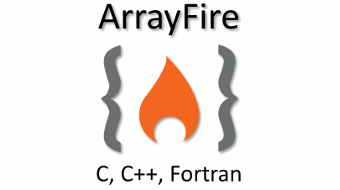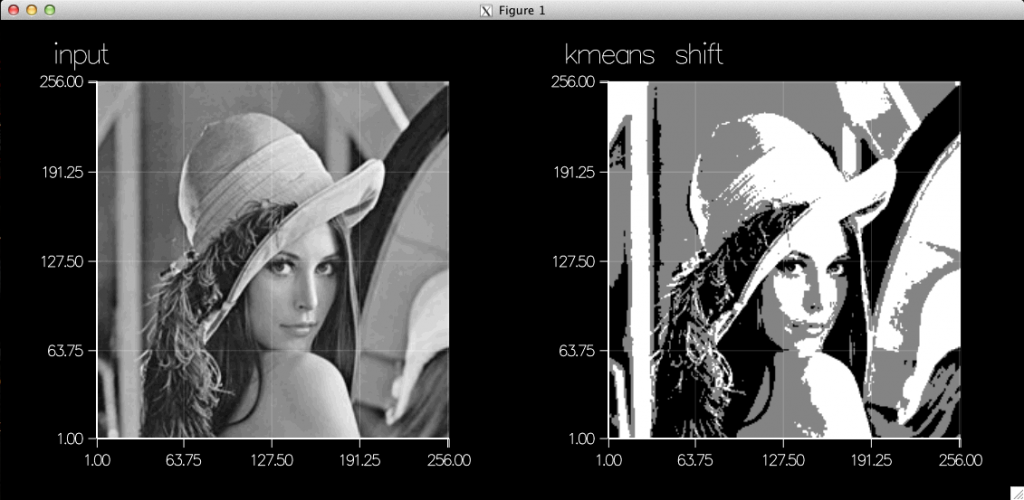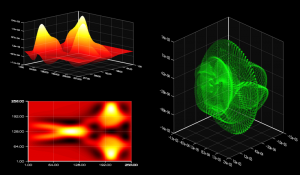# Do More, Code Less with ArrayFire GPU Matrix LibraryThis is a guest post by Chris McClanahan from ArrayFire (formerly AccelerEyes).

ArrayFire is a fast and easy-to-use GPU matrix library developed by ArrayFire. ArrayFire wraps GPU memory into a simple “array” object, enabling developers to process vectors, matrices, and volumes on the GPU using high-level routines, without having to get involved with device kernel code.

# ArrayFire Feature Highlights

• ArrayFire provides a high-level array notation and an extensive set of functions for easily manipulating N-dimensional GPU data.
• ArrayFire provides all basic arithmetic operations (element-wise arithmetic, trigonometric, logical operations, etc.), higher-level primitives (reductions, matrix multiply, set operations, sorting, etc.), and even domain-specific functions (image and signal processing, linear algebra, etc.).
• ArrayFire can be used as a self-contained library, or integrated into and supplement existing CUDA code. The array object can wrap data from CUDA device pointers and existing CPU memory.
• ArrayFire contains built-in graphics functions for data visualization. The graphics library in ArrayFire provides easy rendering of 2D and 3D data, and leverages CUDA OpenGL interoperation, so visualization is fast and efficient. Various visualization algorithms make easy to explore complex data.
• ArrayFire offers a unique “gfor” construct that can drastically speed up conventional “for” loops over data. The gfor loop essentially auto-vectorizes the code inside, and executes all iterations of the loop simultaneously.
• ArrayFire supports C, C++, and Fortran on top of the CUDA platform.
• ArrayFire is built on top of a custom just-in-time (JIT) compiler for efficient GPU memory usage. The JIT back-end in ArrayFire automatically combines many operations behind the scenes, and executes them in batches to minimize GPU kernel launches.
• ArrayFire strives to include only the best performing code in the ArrayFire library. This means that the ArrayFire library uses existing implementations of functions when they are faster—such as Thrust for sorting, CULA for linear algebra, and CUFFT for fft.

# K-means Clustering: An ArrayFire Example

The K-means clustering algorithm is a popular method of cluster analysis commonly used in data mining and machine learning applications. The goal of the algorithm is to partition n data points into k clusters in which each observation belongs to the cluster with the nearest mean. K-means can also be used in image processing applications as a method for image segmentation. Here is some pseudocode for the K-means clustering algorithm.

```Choose k data points to act as initial cluster centers
Until the cluster centers do not change:
Assign each data point (pixel) to the nearest (color-distance) cluster
Update the cluster centers with the average of the pixels in the current cluster
End```

Implementing k-means in raw CUDA C/C++ can be a daunting task; even using Thrust it takes over 300 lines of code. With ArrayFire, a k-means implementation can be written in about 10 times fewer lines of code than an equivalent Thrust implementation, allowing the programmer to spend time and effort on high-level algorithms rather than low-level implementation details. The following code snippet is from the K-Means example (included with ArrayFire), which demonstrates easy k-means image segmentation.

```// kmeans(input,input,output)
//  data:   input,  1D or 2D (range [0-1])
//  k:      input,  # desired means (k > 1)
//  means:  output, vector of means
void kmeans(array& data, int k, array& means) {

array datavec = flat(data);              // convert 2D -> 1D
float minimum = min(datavec);            // get minimum value
datavec = datavec - minimum;             // re-center range

int nbins = max(datavec) + 1;            // number of color bins
array means_vec = array(seq(k)) * nbins / (k + 1); // initial centroids

array hist_counts = zero(1, nbins);      // initialize histogram bins
array hist = histogram(datavec, nbins);  // compute image histogram

array hist_idx = where(hist);            // get non-zero histogram bins
int num_uniq = hist_idx.elements();      // number of non-zero bins

while (1) {                              // convergence loop
array prev_means = means_vec;          // running means total

gfor(array i, num_uniq) {              // update all bins in parallel
array diffs = abs(hist_idx(i) - means_vec); // current classifications
array val, idx;
min(val, idx, diffs);                // get index of minimum value
hist_counts(hist_idx(i)) = idx;      // update bins
}

for (int i = 0; i < k; ++i) {
array m = where(hist_counts == i);   // find all occurrences
means_vec(i) = sum(m * hist(m)) / sum(hist(m)); // recalculate means
}

if (norm(means_vec - prev_means) < 1) break; // stop when converged
}

means = means_vec + minimum;             // re-center range, output means
}```

This heavily commented code sample uses several of the features mentioned previously; notice how expressive and powerful the ArrayFire syntax can be. The complete demo source code also demonstrates how to re-color an image based on the computed means (a “k-means shift”). The following text and image are the output of running the k-means example.

```machine_learning \$ ./kmeans
** ArrayFire K-Means Demo **
k = 3
min 29
max 209
means =
69.2678
125.1634
173.2486```A relative of the k-means clustering algorithm is mean-shift clustering, in which local clusters form based on the local data, and not based on a specific k. ArrayFire also includes a meanshift() function for simple image filtering.

# Beautiful ArrayFire Graphics

The “Shallow Water Equations” (SWE) example included with ArrayFire demonstrates how to use ArrayFire to simulate and visualize the shallow water equations on the GPU. It makes heavy use of convolution and element-wise arithmetic, and showcases 2D and 3D graphics rendering, as you can see in the following screenshot. The upper left surface plot and bottom left image plot show the current wave formation, and the 3D points on the right plot gradient vs. magnitude.# Easy Integration with CUDA

ArrayFire can easily share data with existing CUDA device memory, allowing plug-in functionality to existing CUDA applications. The following code snippet demonstrates how to use ArrayFire with a CUDA device memory pointer for image edge detection.

```// cuda_img_ptr is an existing cuda device memory pointer
...
// 3x3 sobel weights
const float h_sobel[] = {
-2.0, -1.0,  0.0,
-1.0,  0.0,  1.0,
0.0,  1.0,  2.0
};
array sobel_k = array(3, 3, h_sobel);
// wrap cuda memory in an ArrayFire array object
array image = array(m, n, cuda_img_ptr, afDevice);
// run filter to get edges
array edges = convolve(image, sobel_k);
// convert array memory back to a raw cuda device pointer
cuda_img_ptr = edges.device();
// continue using cuda_img_ptr
...```

# Multi-GPU Support

You can use ArrayFire to easily parallelize computation among multiple CUDA devices. The deviceset() function is used to select a device on which subsequent operations will be performed. The following example shows how to run an FFT across many devices in parallel.

```// divide up work across all GPUs
array *y = new array[num_gpus];
for (int i = 0; i < num_gpus; ++i) {
deviceset(i);             // change GPU
array x = randu(5,5);     // add data to selected GPU
y[i] = fft(x);            // put work in queue
}
// all GPUs are now computing simultaneously, until done```

See the multi-gpu gemv code included with ArrayFire for a more complete distributed-computing example. Using deviceset() in combination with gfor offers massive parallelism with minimal code complexity.

# Limitations of ArrayFire

While ArrayFire has a solid set of routines for 1D/2D/3D data, it does not offer true N-D support. ArrayFire is currently limited to 4-dimensional data, and most functions only support up to 3D arrays.

Currently, not every function supports gfor. While many functions can be used inside gfor loops, there are some that cannot. Also, gfor currently requires each iteration to be independent, which precludes certain types of computation. Over time, however, ArrayFire hopes to enable gfor support for all computations.

# Gear Up for Speed Up

ArrayFire is a fast matrix library for GPU computing with an easy-to-use API. Its “array”-based function set makes GPU programming simple and accessible. ArrayFire is cross-platform (Linux,Windows,OSX) and offers hundreds of routines in areas of matrix arithmetic, signal processing, linear algebra, statistics, image processing, and can easily integrate into existing CUDA applications. Read more about ArrayFire and try out our library for free!

Chris McClanahan is a software engineer at ArrayFire.